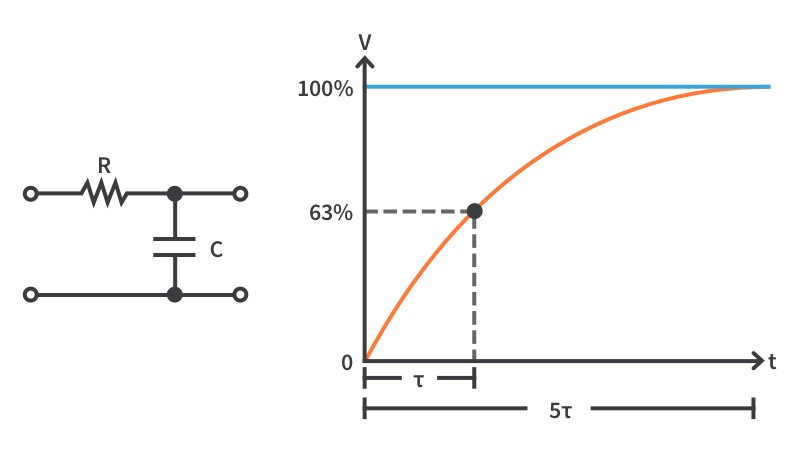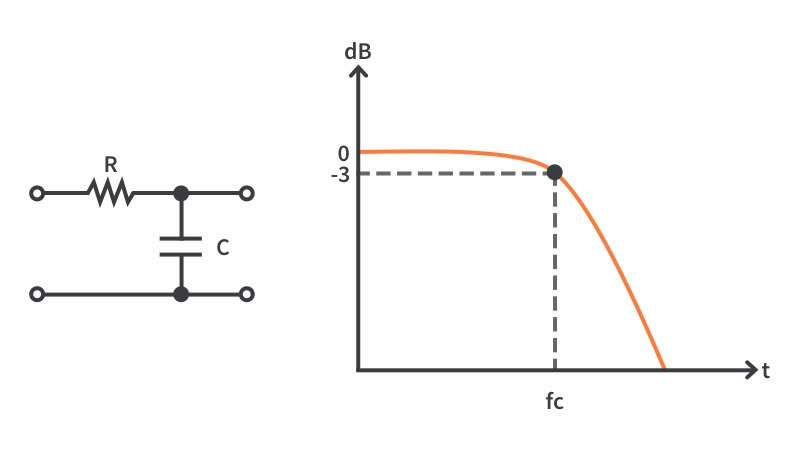# RC CalculatorInputOutput
V

An RC circuit is an extremely common circuit configuration, one that you can find by itself or, more frequently, as part of a bigger circuit. Also, sometimes RC circuits are unintentional and simply parasitic in nature. With this calculator, you can get an intuitive understanding of what happens with a charging and discharging RC circuit in the time domain.

With only the values of the resistor and capacitor, we can find the time constant of the RC circuit, also known as tau, which is the amount of time required to charge or discharge a capacitor in series with a resistor to 63.2% of the initial charge voltage. For example, if you are charging from 0 to 10 volts, then the time constant is how long it takes to reach 6.32 volts. The capacitor charge time, to become fully charged, is considered to be 5 time constants.InputOutput
Hz